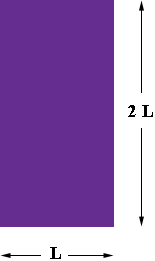SEARCH HOMEMath Central Quandaries & QueriesQuestion from Hayley, a student: A rectangles width is exactly twice its length. The area of the rectangle is 450 square feet. What are the length and width of the rectangle?Hi Hayley,

Draw a diagram.I let the length of the rectangle be $L$ feet then the width must be $2 L$ feet. The area of a rectangle is the length times the width so $2 L \times L = 450$ square feet. Solve for $L.$

PennyMath Central is supported by the University of Regina and The Pacific Institute for the Mathematical Sciences.